### Home > A2C > Chapter 11 > Lesson 11.3.1 > Problem11-134

11-134.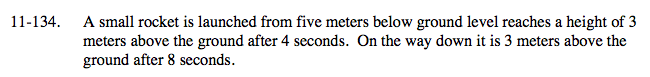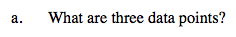Define the variables; x = time, y = height.

(0, −5), (4, 3), (8, 3)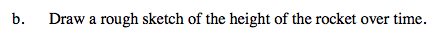See part (a).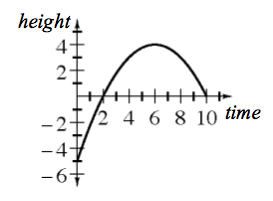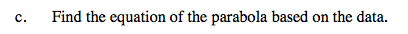Use the points from (a) and the general equation y = ax2 + bx + c.

$y=-\frac{1}{4}x^{2}+3x-5$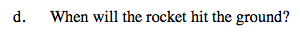See the graph from part (b).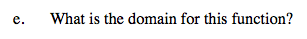See the graph from part (b).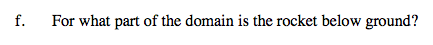See the graph from part (b).